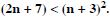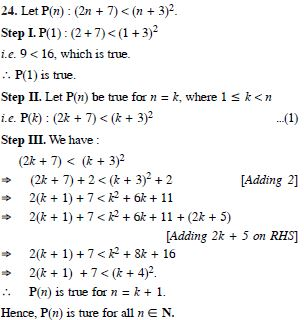# NCERT Solutions for Class 11 Math Chapter 4 - Mathematical Induction

Practice your Maths skills with Free NCERT Solutions at Aasoka. Prepared by the subject matter experts, these solutions help students score the marks they desire. Students can also prepare for competitive exams such as JEE and NEET with the help of NCERT Solutions for Class 11. These solutions come in handy when students want to enhance their skills. The questions and answers are as per the latest CBSE syllabus which makes it easier for students to cover the entire chapter.

The “Mathematical Induction” chapter of Maths Class 11 covers problems and applications related to the principle of mathematical induction, the process of providing induction, deduction, and much more.

##### Question 1: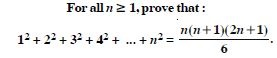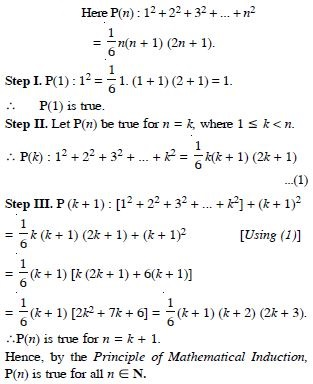##### Question 2: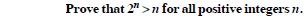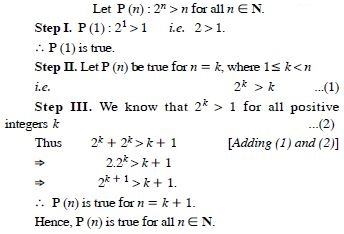##### Question 3: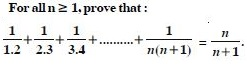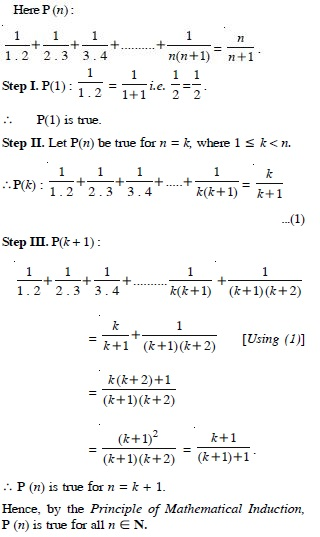##### Question 4: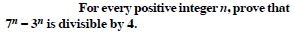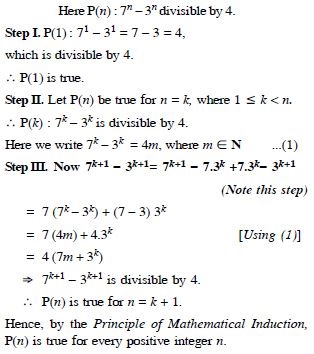##### Question 5: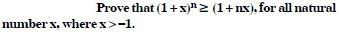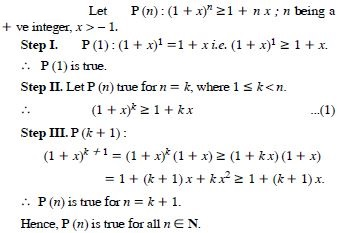##### Question 6: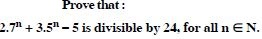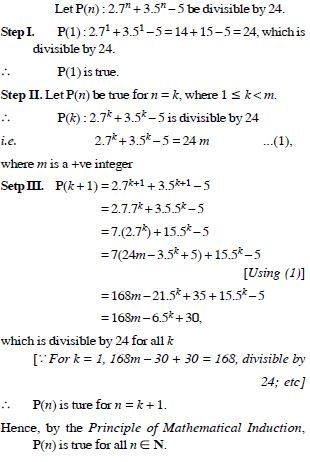##### Question 7: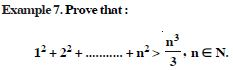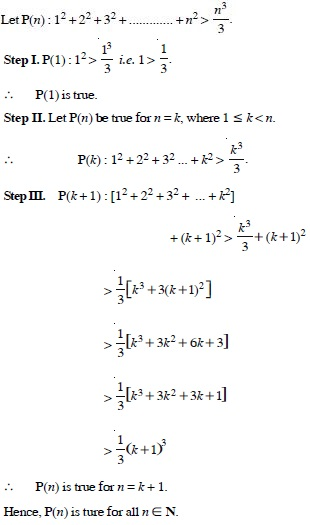##### Question 8: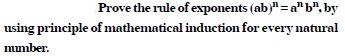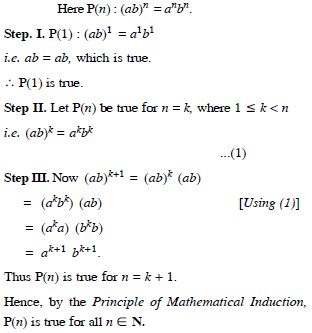##### Question 9:

Prove the following by using principle of mathematical induction for all n $\in$ N :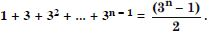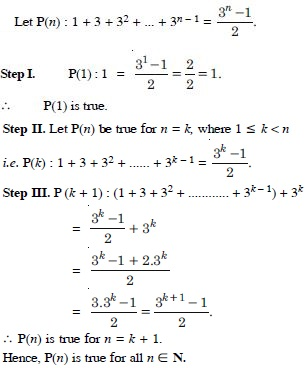##### Question 10:

Prove the following by using principle of mathematical induction for all n $\in$ N :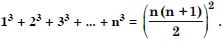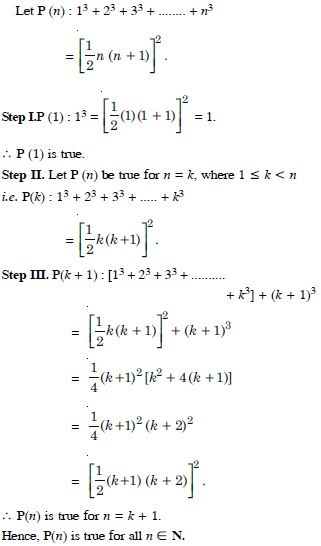##### Question 11:

Prove the following by using principle of mathematical induction for all n $\in$ N :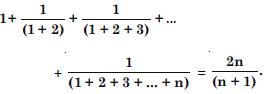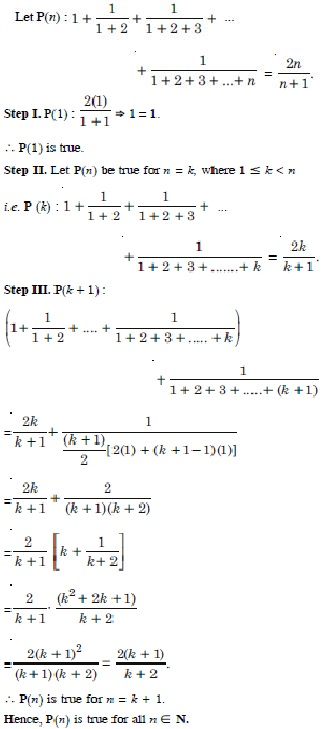##### Question 12:

Prove the following by using principle of mathematical induction for all n $\in$ N :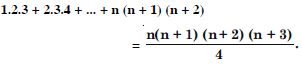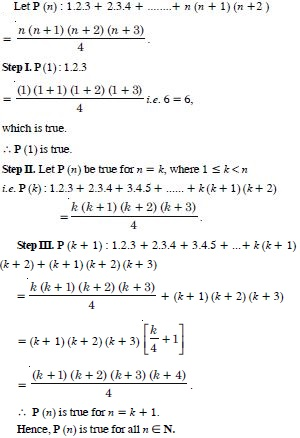##### Question 13:

Prove the following by using principle of mathematical induction for all n $\in$ N :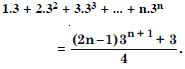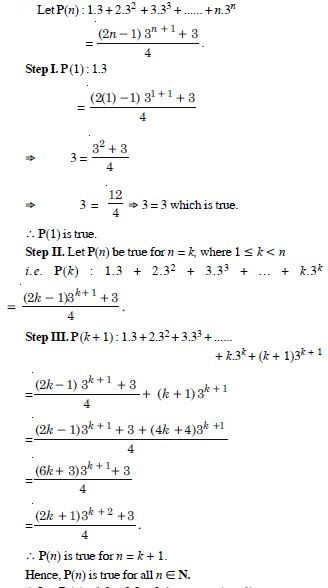##### Question 14:

Prove the following by using principle of mathematical induction for all n $\in$ N :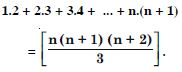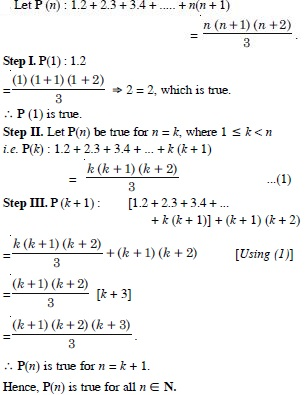##### Question :15

Prove the following by using principle of mathematical induction for all n $\in$ N :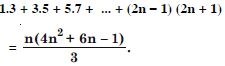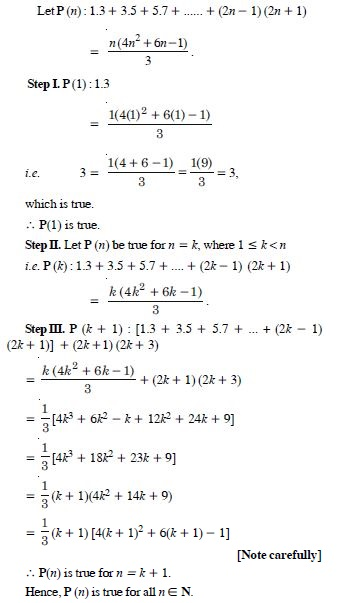##### Question 16:

Prove the following by using principle of mathematical induction for all n $\in$ N :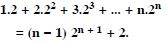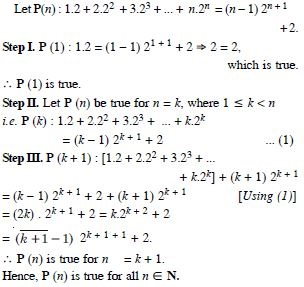##### Question 17:

Prove the following by using principle of mathematical induction for all n $\in$ N :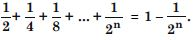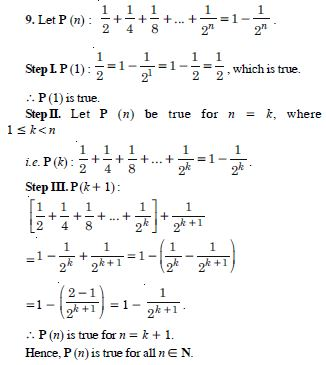##### Question 18:

Prove the following by using principle of mathematical induction for all n $\in$ N :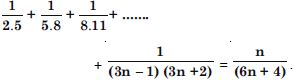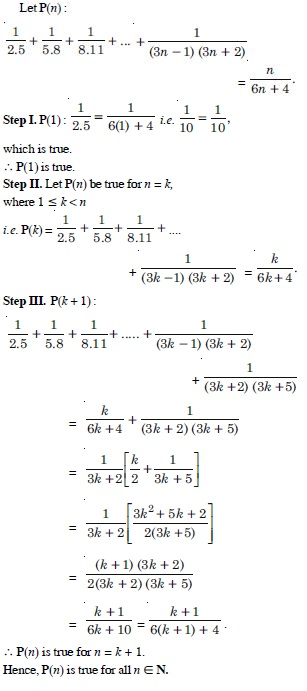##### Question 19:

Prove the following by using principle of mathematical induction for all n $\in$ N :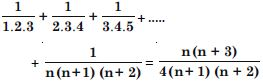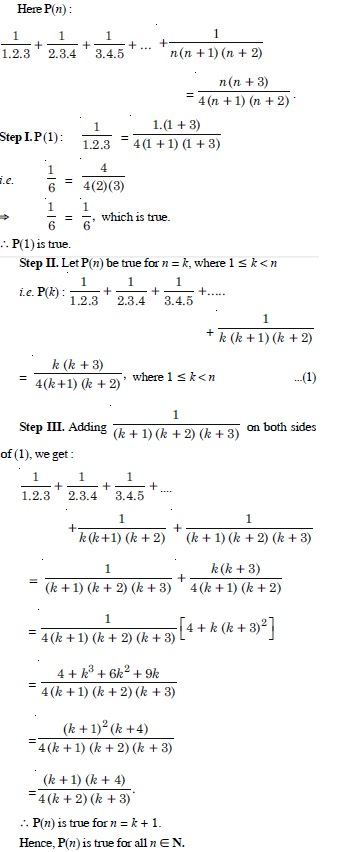##### Question 20:

Prove the following by using principle of mathematical induction for all n $\in$ N :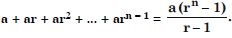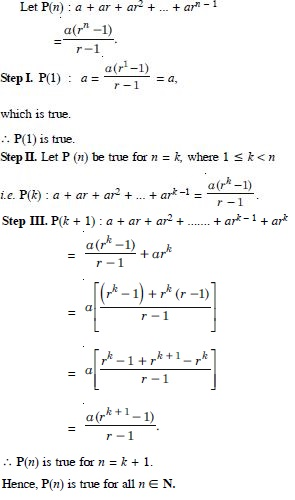##### Question 21:

Prove the following by using principle of mathematical induction for all n $\in$ N :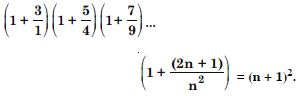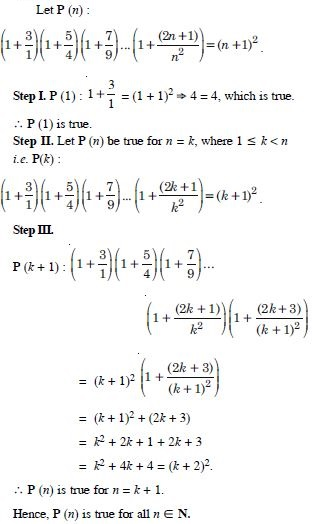##### Question 22:

Prove the following by using principle of mathematical induction for all n $\in$ N :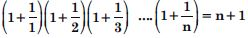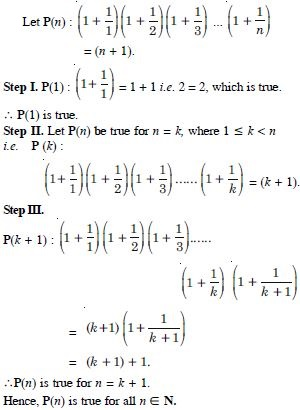##### Question 23:

Prove the following by using principle of mathematical induction for all n $\in$ N :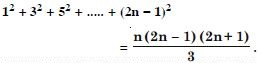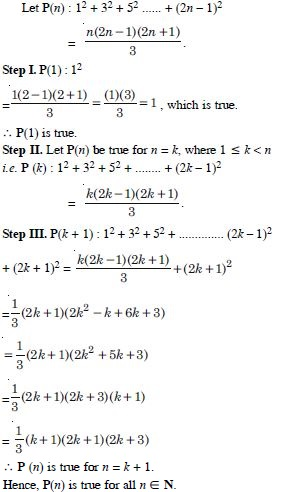##### Question 24:

Prove the following by using principle of mathematical induction for all n $\in$ N :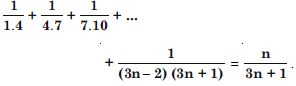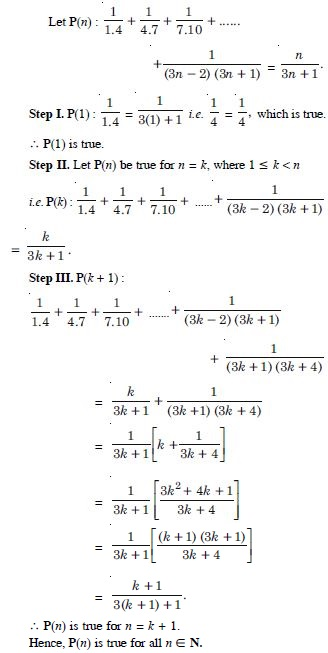##### Question 25:

Prove the following by using principle of mathematical induction for all n $\in$ N :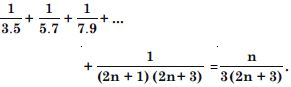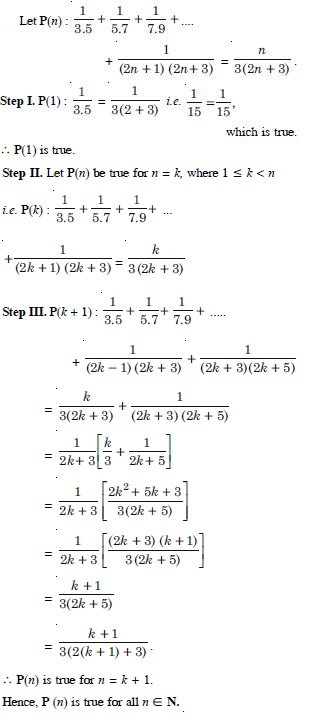##### Question 26:

Prove the following by using principle of mathematical induction for all n $\in$ N :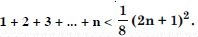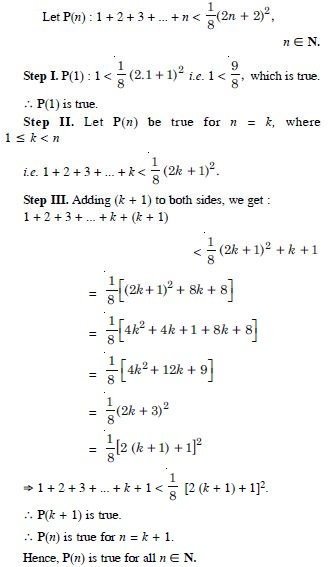##### Question 27:

n (n + 1) (n + 5) is a multiple of 3.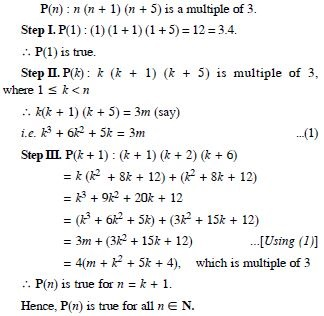##### Question 28:

Prove the following by using principle of mathematical induction for all n $\in$ N :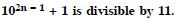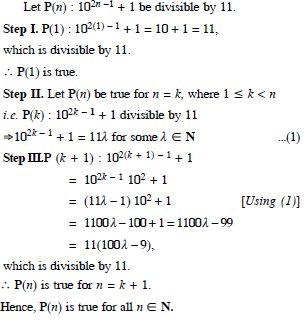##### Question 29:

Prove the following by using principle of mathematical induction for all n $\in$ N :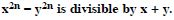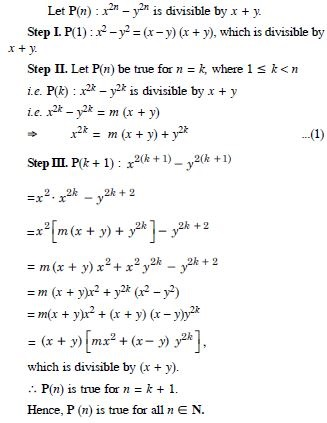##### Question 30:

Prove the following by using principle of mathematical induction for all n $\in$ N :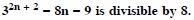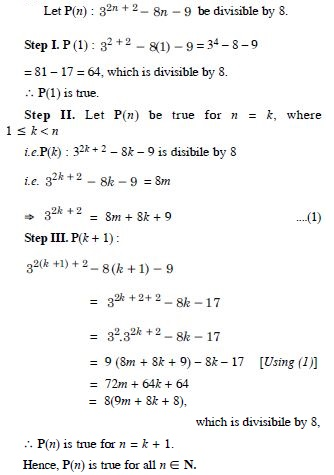##### Question 31:

Prove the following by using principle of mathematical induction for all n $\in$ N :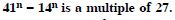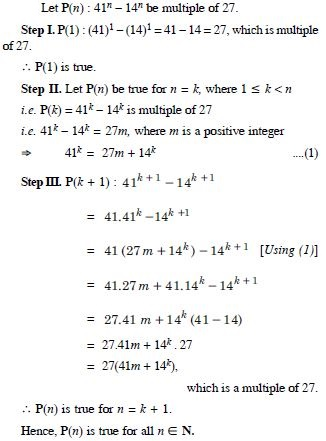Prove the following by using principle of mathematical induction for all n $\in$ N :